# The Triangular Hebesphenorotundaeic Rhombochoron

The triangular hebesphenorotundaeic rhombochoron, or J92 rhombochoron for short, is a 4D CRF polychoron whose surface consists of 4 triangular hebesphenorotunda (J92), 6 metabidiminished icosahedra, 6 triangular prisms, 24 pentagonal pyramids, 30 square pyramids, and 12 tetrahedra, for a total of 82 cells, 262 polygons (206 triangles, 30 squares, 2 hexagons, 24 pentagons), 246 edges, and 66 vertices. It was discovered on 6 February, 2014, as part of the ongoing project to find all 4D CRF polytopes (generalizations of the Johnson solids to 4D), and was the first non-trivial example of a CRF polychoron that has J92 (triangular hebesphenorotunda) cells. Prior to this, the only such polytope known was the J92 prism.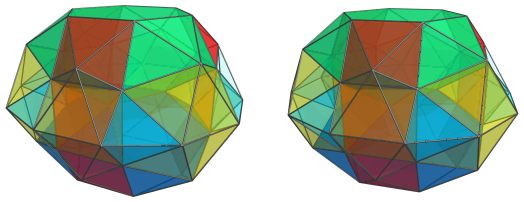Previously, it was thought to be very difficult to build CRF polytopes out of the Johnson solids toward the end of Norman Johnson's list, including the triangular hebesphenorotunda (J92), due to their unusual shapes. Polytopes containing odd-shaped cells like J92 were expected to be unlikely to close up in a CRF way, besides the trivial construction of their prisms. Two days prior to the discovery of the J92 rhombochoron, however, a CRF polychoron with icosahedral symmetry was discovered that featured bilunabirotunda (J91) as cells, dubbed the castellated rhombicosidodecahedral prism. This suggested that it might be possible to construct non-trivial CRF polychora with these unusual Johnson solids after all, especially the ones that showed some connection with the icosahedron, like J91 and J92. Sure enough, the J92 rhombochoron was discovered shortly after, followed by numerous other 4D CRF polytopes that contain either of these two Johnson solids as cells, sometimes both.

## Structure

Due to the unusual structure of the J92 rhombochoron, it is helpful to examine it from various different 4D viewpoints. Our first look will be its parallel projections centered on the triangular face shared by a pair of J92 cells:

### Centered on J92 Top Triangle

#### The Near Side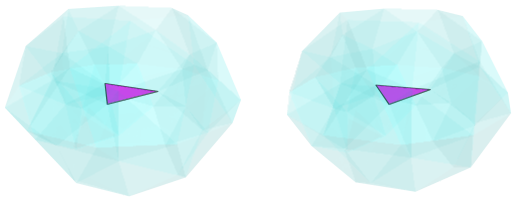The above image shows the nearest triangular ridge to the 4D viewpoint. For clarity, we render all other elements in a light transparent color.

This triangular ridge is where two J92 cells meet: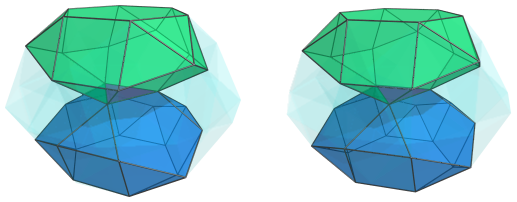Around the edges of the triangular ridge are three metabidiminished icosahedra (J62):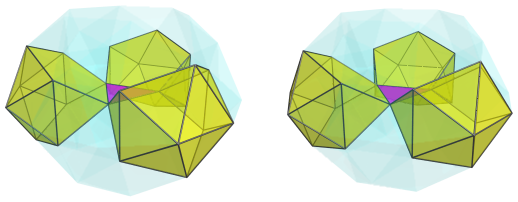For clarity, we have omitted the J92 cells. These J62 cells serve as wedge-shaped surfaces interfacing the pentagonal faces of the J92 cells. They alternate with 3 square pyramids that touch the triangular ridge's vertices, as shown next: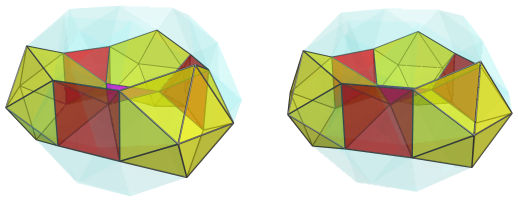The following image shows all the cells so far together: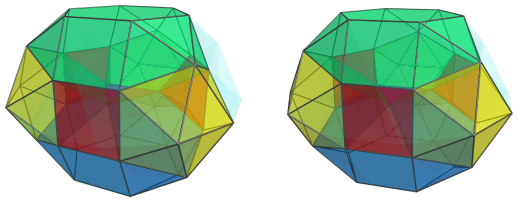The bases of the square pyramids are joined to 3 triangular prisms: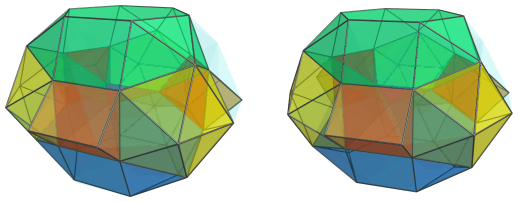The triangular faces of these triangular prisms are joined to 6 tetrahedra that link them to the metabidiminished icosahedra: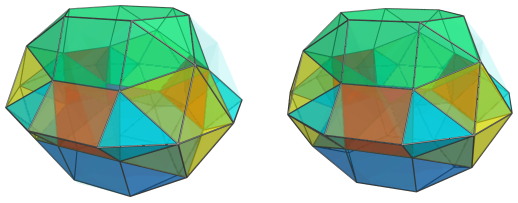The remaining square faces of these triangular prisms are joined to 6 more square pyramids: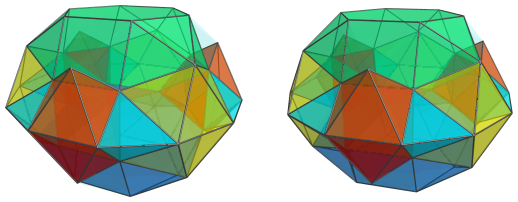Another set of 6 square pyramids link these to the square faces of the J92 cells: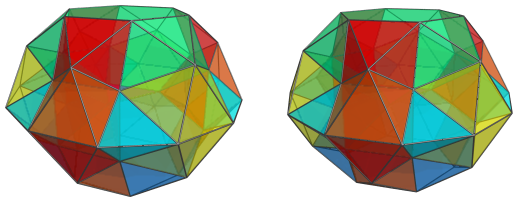The pentagons that have now become obvious are where 12 pentagonal pyramids fit in, linking together the pairs of square pyramids, the J92 cells, the tetrahedra, and the J62 (metabidiminished icosahedron) cells.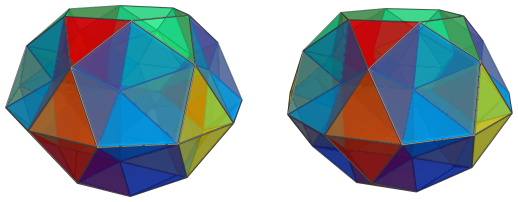The following image shows these pentagonal pyramids with other cells rendered in a light transparent color, for better clarity: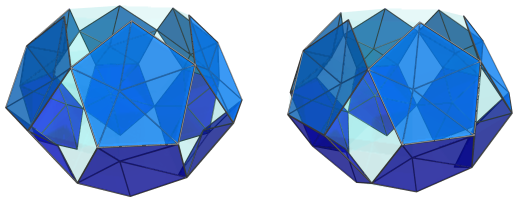#### The Far Side

The 12 pentagonal pyramids on the near side of the J92 rhombochoron are paired up with another 12 pentagonal pyramids on the far side, shown below: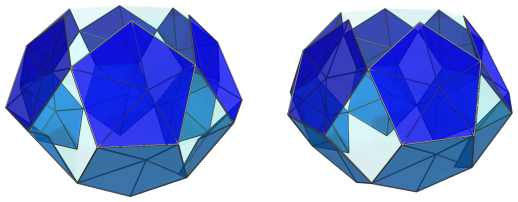It may not be immediately obvious, but the apices of these far-side pentagonal pyramids are bent in the opposite direction from their near-side counterparts. This is because the arrangement of cells on the far side of the polytope, while mirroring the arrangement on the near side, is rotated by 60°, and is thus in a gyrated orientation. This is further indicated by the orientation of the two J92 cells on the far side, shown below: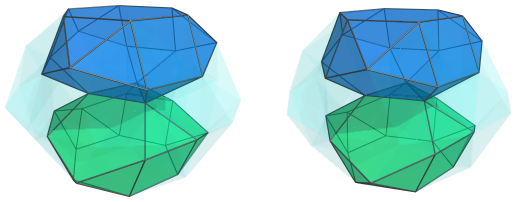We see that this is the same arrangement as before, but rotated by 60°. Again, 3 square pyramids link the adjacent triangles of the J92 cells: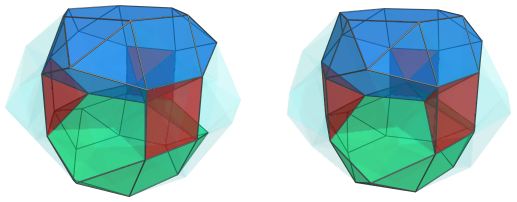The gaps between the pentagons of the two J92 cells are filled with 3 J62 (metabidiminished icosahedron) cells: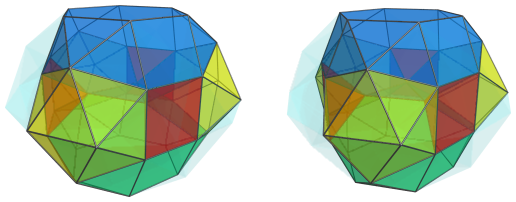The square bases of the square pyramids are joined to 3 triangular prisms, just like on the near side: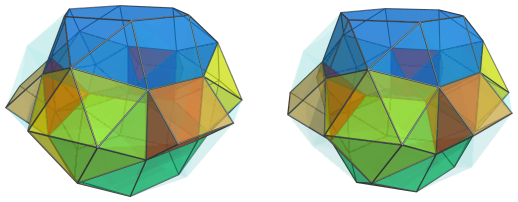The triangular faces of these triangular prisms are, of course, joined to 6 tetrahedra that sit between them and the metabidiminished icosahedra: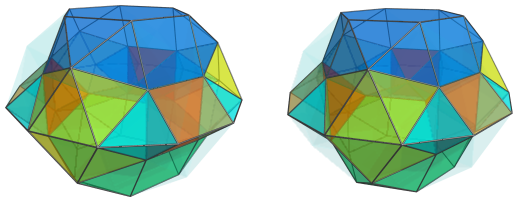Just like on the near side, the square faces of the triangular prisms are joined to pairs of square pyramids that link them to the J92 cells: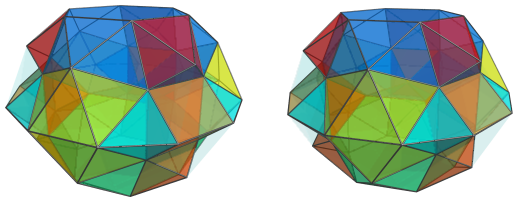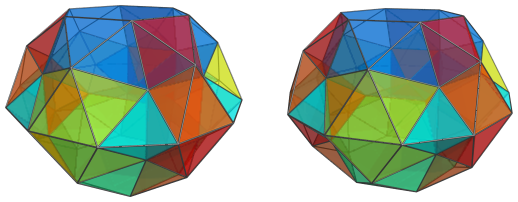Finally, there are the 12 pentagonal pyramids that we saw earlier: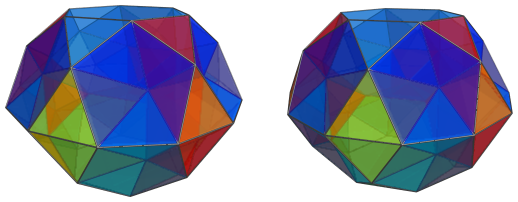#### Summary

The following table summarizes the cell counts of the J92 rhombochoron according to the 4D viewpoint centered on the triangle shared by two J92 cells:

Region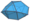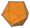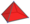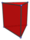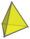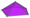Near side 2 3 3 + 2*(3+3) = 15 3 6 12
Far side 2 3 15 3 6 12
Total 4 6 30 6 12 24

### Centered on J92 Hexagon

Now we look at the J92 rhombochoron from a slightly different angle, this time centered on a hexagonal face between two J92 cells.

#### The Near Side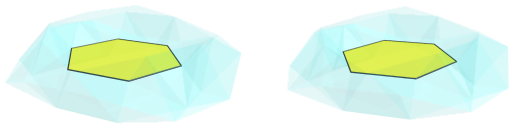The above image shows the nearest hexagonal ridge to the 4D viewpoint. This ridge is where two J92 cells meet: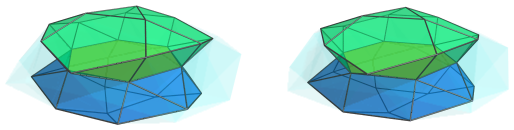The angle between these two cells is 60°, which is rather sharp. This indicates that the J92 rhombochoron is a rather flat object, which can also be seen in the flatness of its projection from this viewpoint. The previous projections were looking at the flatter portions of the polytope, like looking at a lenticular shape face-on; whereas now we are looking at it from the side, like looking at a lenticular shape edge-wise.

The 3 square faces of each of these J92 cells are joined to square pyramids that link them to the other cell, for a total of 6 square pyramids: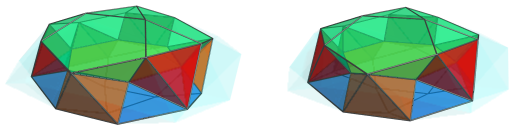The remaining gaps between the two J92 cells are filled in by 6 pairs of pentagonal pyramids: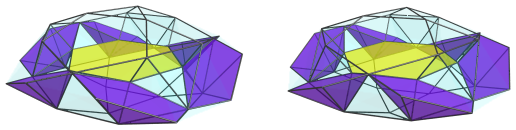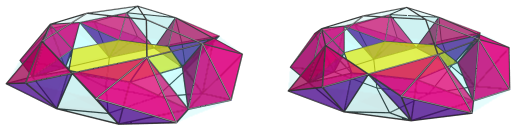For clarity, we have omitted the previous cells, and only left the nearest hexagon for reference. The next image shows all these cells together: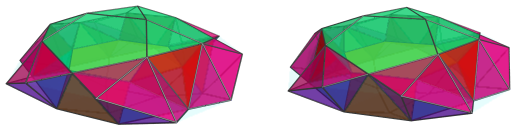As the now-obvious gaps indicate, there are another 6 square pyramids that lie over the previous ones, fitting between every two adjacent pairs of pentagonal pyramids: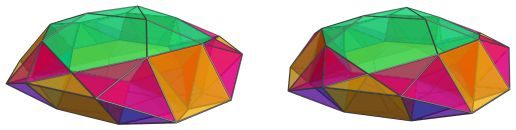These are all the cells that lie on the near side of the J92 rhombochoron from this viewpoint. Past this point, we reach the “equator”, or the limb, where the cells lie at a 90° angle to the 4D viewpoint.

#### The Equator

There are 6 metabidiminished icosahedra that lie on the equator: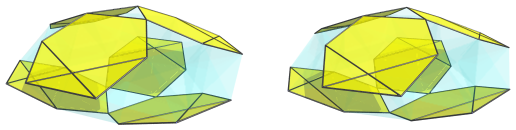For clarity, we have omitted the cells that we have seen previously. These cells appear flattened into hexagons because they lie at a 90° angle to the 4D viewpoint. In 4D, they are full-bodied metabidiminished icosahedra.

The astute reader may have noted from the triangle-centered projections from before that the pairs of adjacent square pyramids that connect to the square faces of the J92 cells join to triangular prisms at the other end. From the current hexagon-centered viewpoint, these triangular prisms lie exactly on the equator; they are shown below: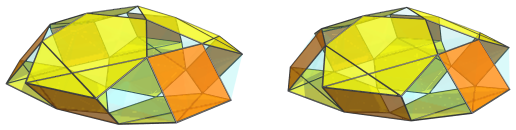They appear foreshortened into squares here because they lie at a 90° angle to the 4D viewpoint. In 4D they are perfectly uniform triangular prisms. The edges of these squares opposite where they meet the metabidiminished icosahedra are actually the projections of one of the square faces of the triangular prisms. These square faces are joined to 6 square pyramids that are wedged between adjacent metabidiminished icosahedra: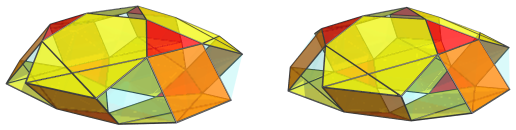The remaining edges of the square projections of the triangular prisms are the triangular faces of these prisms; they are joined to tetrahedra on either side: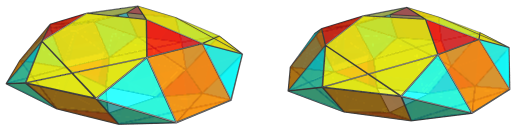Note that each cyan triangle in the above image is the projection of one tetrahedron; so each pair of triangles represents two tetrahedra sharing an edge. These tetrahedra have been foreshortened into triangles because they are at a 90° angle to the 4D viewpoint; in 4D space, they are perfectly regular tetrahedra.

These are all the cells that lie on the equator of the J92 rhombochoron. Past this point, we reach the far side of the polytope, where the arrangement of cells is exactly the same as the near side. The remaining triangular gaps at the top and bottom are not images of any cells; they are where the two J92 cells on the near side touch their counterparts on the far side.

#### Summary

The following table summarizes the cell counts of the J92 rhombochoron, as seen from the hexagon-centered projections:

RegionNear side 2 0 6 + 6 = 12 0 0 12
Equator 0 6 6 6 12 0
Far side 2 0 12 0 0 12
Total 4 6 30 6 12 24

## Coordinates

The Cartesian coordinates of the 66 vertices of the J92 rhombochoron, with edge length 2 and centered on the origin, are:

 ±(0, 2/√3, φ2/√3, 0) ±(±1, -1/√3, φ2/√3, 0) ±(±1, φ3/√3, φ/√3, ±1) ±(±φ2, -1/(φ√3), φ/√3, ±1) ±(±φ, -(φ+2)/√3, φ/√3, ±1) ±(±φ2, φ2/√3, 1/√3, ±φ) ±(0, -2φ2/√3, 1/√3, ±φ) (±1, ±√3, 0, ±φ2) (±2, 0, 0, ±φ2) (±φ2, (φ+3)/√3, 1/(φ√3), 0) (±φ2, -(φ+3)/√3, -1/(φ√3), 0) (±1, (3+2φ)/√3, -1/(φ√3), 0) (±1, -(3+2φ)/√3, 1/(φ√3), 0) (±(φ+2), φ/√3, 1/(φ√3), 0) (±(φ+2), -φ/√3, -1/(φ√3), 0)

where φ=(1+√5)/2 is the Golden Ratio.

Last updated 18 Jun 2019.